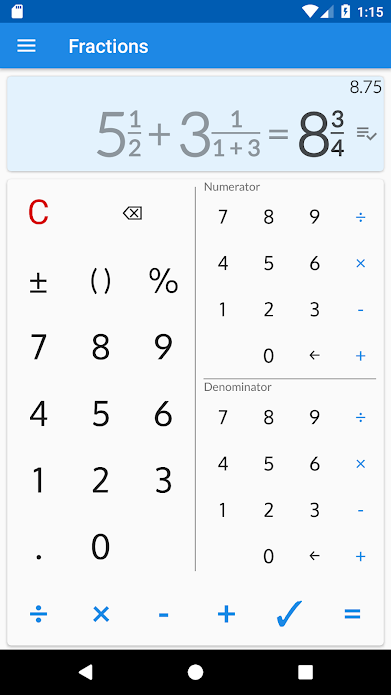I don’t know how I ever did without this calculator. How many times have you ever had to add fractions together? If, like me, not that often; but, when I do have to perform fraction calculations I find it a challenge. This calculator app, called Fractions Calculator, eliminates this problem; plus, it has taught me about fractions.Best and free offline fraction calculator ✌, supporting also decimals, percentages, and parentheses that shows a detailed solution for all calculations. 😊 At school 🏫 or home 🏠 need to solve simple or complex math fraction problems, convert fractions into decimals, or decimals into fractions? – this fraction calculator will do it ✅ and will show how it was done 😺 Perfect for students, parents, teachers, and woodworkers to solve or verify fraction everyday problems. Features of this fraction calculator free app ➕ ➖ ❌ ➗ 🍉 Supports combining decimal and fractional numbers. Automatically reduces fraction result to its simplest form. 🍇 Converts fractions into decimals and decimals into fractions. Calculated result is displayed using fractions. Equivalent value in decimals is also available. 🍌 Shows a detailed solution, step by step for all fraction problems and calculations. 🍎 History of previous calculations is available. 🍒 Besides basic math operations such as add, subtract, multiply and divide; fraction calculator allows calculating percentages for fractions. 🍅 Triple keypad available 🍍 Works with big numbers. No limits 😋 We have made this fraction calculator a